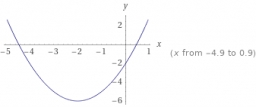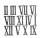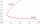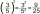# The sum 16

The sum of A and B is 36. The difference of A & B is 8. find the larger number of A.

A =  22

### Step-by-step explanation:Did you find an error or inaccuracy? Feel free to write us. Thank you!Tips to related online calculators
Do you have a system of equations and looking for calculator system of linear equations?

## Related math problems and questions:

• The difference 2The difference between the two numbers is 25. The smaller number is 1/6th of the larger number. What is the value of the smaller number?
• The sum 8The sum of two numbers is 21. If three times the smaller numbers is two less than twice the larger number, find the two numbers.
• The largerThe larger of two numbers is nine more than four times the smaller number. The sum of the two numbers is fifty-nine. Find the two numbers.
• The sum 4The sum of Robin's age is 45. Seven years ago, Robin was 16 years more than one half as old as Bruno then. How old is Bruno?
• Two numbers 7The sum of two consecutive even numbers is 30. Find the numbers.
• The differenceThe difference of two numbers is 1375. If their exact quotient is 12. Find the two numbers
• Speed of Slovakian trainsRudolf decided to take the train from the station 'Ostratice' to 'Horné Ozorovce'. In the train timetables found train Os 5409 : km 0 Chynorany 15:17 5 Ostratice 15:23 15:23 8 Rybany 15:27 15:27 10 Dolné Naštice 15:31 15:31 14 Bánovce nad Bebravou 15:35 1
• The sum 9The sum of two integers is 4. Their difference is 8. What are this two integers?
• A number 4A number is made up of two digits. The sum of the digits is 11. if the digits are interchanged, the original number is increase by 9. Find the original number
• Sum and productThe sum of the real numbers x and y is 12. Their difference is 8. What is the value of xy?
• Find twoFind two consecutive natural numbers whose product is 1 larger than their sum. Searched numbers expressed by a fraction whose numerator is the difference between these numbers and the denominator is their sum.
• Unknown number 716% of the unknown number is by 21 less than unknown number itself. Determine the natural unknown number.
• Ratio of two unknown numbersTwo numbers are given. Their sum is 30. We calculate one-sixth of a larger number and add to both numbers. So we get new numbers whose ratio is 5:7. Which two numbers were given?
• Comparing exponentsA) 8 (6²)(8²) b) 16 (6²)(8²) c) (6¹+²) (8²)
• Digit sumThe digit sum of the two-digit number is nine. When we turn figures and multiply by the original two-digit number, we get the number 2430. What is the original two-digit number?
• Two numbersThe difference between the two numbers is 74. If we divide a larger number by a smaller one, we get a quotient 7 and the rest of 2. Determine both numbers.
• Two unknown numbersOne number is 2 less than a second number. Twice the second number is 14 less than 5 times the first. Find the two numbers.